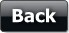All exams are online now due to COVID related precautions | Download the app or write to us at info@vskills.in

# Learning Resources## Formula

Formula
It is the most used facility of MS-EXCEL. A formula is a cell entry that calculates values to return a result and it begins with the equal sign (=) and uses values or cell references and operators or MS-Excel in-built function.

Operators
Various operators are

Operator

What It Does

+

Addition

-

Subtraction

*

Multiplication

/

Division

=

Equal to

Less than

<=

Less than or equal to

Greater than

>=

Greater than or equal to

<>

Not equal to

%

Percentage

^

Exponentiation

Functions
Functions are prewritten formulas that perform certain types of calculations automatically.
For example the function used for today date is TODAY (). Functions are used as
• Using Functions Wizard to create formulae
• Directly Inserting Functions
Cells address used in formulae can be absolute or relative